# Key Exchange Standards

## From Real-World Cryptography by David Wong

In this article, the author teaches readers about the Diffie-Hellman key exchange standard, which was the very first key exchange algorithm ever invented, and the Elliptic Curve Diffie-Hellman key exchange standard, which is the Diffie-Hellman built with elliptic curves.

• The second key exchange algorithm I will talk about is actually the same Diffie-Hellman algorithm but built with elliptic curves, it is thus usually called Elliptic Curve Diffie-Hellman (ECDH).

## Diffie-Hellman (DH)

In 1976, Whitfield Diffie and Martin E. Hellman wrote their seminal paper on the DH key exchange algorithm entitled “New Direction in Cryptography” (what a title right?).

1. An operation defined on these elements that satisfy certain properties.
• 54 = 2 mod 13
• 170 = 0 mod 17
• and so on…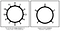Figure 1. The group of integers modulo the prime number 5 can be pictured as a clock that resets to 0 after the number 4. Thus 5 is represented as 0, 6 as 1, 7 as 2, 8 as 3, 9 as 4, 10 as 0, and so on.

## Quite straightforward isn’t it?

I said earlier that the operation satisfies a number of properties. One of them is that every element defined in our group must have an inverse. We say that an element a has an inverse b if

• 3 x 3 x 3 as 33
• and so on…
• 42 mod 5 = 1
• 43 mod 5 = 4 (we start again from the beginning)
• 34 mod 5 = 1
• and so on …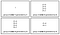Figure 2. The different subgroups of the multiplicative group modulo 5. They all include the number 1 (called the identity element) and have different orders (number of elements).
• A generator can be used to generate a whole (sub)group.

## This is the secret sauce behind Diffie-Hellman!

You now know enough to understand how to generate a keypair in DH:

1. Each participant generates a random number X, this becomes their private key.
2. Each participant derives their public key as gX mod p.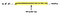Figure 3. Choosing a private key in Diffie-Hellman is like choosing an index in a list of numbers produced by a generator g. The discrete logarithm problem is to find the index from the number alone.
• Bob has a private key b and a public key B = gb mod p.

## Diffie-Hellman Standards

Now that you have seen how Diffie-Hellman works, you understand that participants need to agree on a set of parameters, specifically on a prime number p and a generator g.

## Elliptic Curve Diffie-Hellman (ECDH)

It turns out that the Diffie-Hellman algorithm, which we just discussed, can be implemented in more than just modulo a prime number. All we need is just a group! It also turns out that a group can be made from elliptic curves (a type of curves in mathematics). The idea was proposed in 1985 by Neal Koblitz and Victor S. Miller independently, and much later adopted when Elliptic Curve Cryptography (ECC) (cryptographic algorithms that are based on elliptic curves) started seeing standardization around 2000. The world of applied cryptography quickly adopted algorithms based on elliptic curves as they provided way smaller keys than the previous generation’s algorithms. Compared to the recommended 2048-bit parameters in DH, parameters of 256-bit were possible with the elliptic curve variant of the algorithm.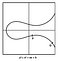Figure 4. One example of an elliptic curve defined by an equation.
1. Draw a vertical line from this newly found point. The vertical line hits the curve in yet another point.
2. This point is the result of adding the original two points together.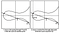Figure 5. An addition operation can be defined over points of an elliptic curve by using geometry!
• What happens if the line we draw in step 1 (or step 2) does not hit the curve at any other point? Well, this is embarassing and we need this special case to work and produce a result. The solution is to define the result as a fictive point (something we made up). This fictive point is called the point at infinity (that we usually write with a big letter O).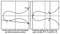Figure 6. Building on figure 5, addition on an elliptic curve is also defined when adding a point to itself, or when two points cancel each other to result in the point at infinity.
• A definition of what an addition means in this set.
• An imaginary point called a point at infinity.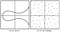Figure 7. Elliptic Curve Cryptography (ECC) in practice is mostly specified with elliptic curves in coordinates modulo a large prime number p. This means that what we use in cryptography looks much more like the right graph than the left graph.
1. Each participant generates a random number X, this becomes their private key.
2. Each participant derives their public key as [X]G.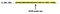Figure 8. Choosing a private key in ECDH is like choosing an index in a list of numbers produced by a generator (or base point) G. The elliptic curve discrete logarithm problem (ECDLP) is to find the index from the number alone.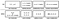Figure 9. Some comparisons between the group used in Diffie-Hellman and the group used in Elliptic Curve Diffie-Hellman.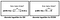Figure 10. A comparison between the discrete logarithm problem modulo large primes, and the discrete logarithm problem in elliptic curve cryptography. They both relate to the Diffie-Hellman key exchange as the problem is to find the private key from a public key.
• Bob has a private key b and a public key B = [b]G.

## Elliptic Curve Diffie-Hellman Standards

The standardization of ECDH has been even more chaotic than DH. Many standardization bodies have come out and specified a large number of different curves, which was then followed by many wars over which curve was more secure. A large amount of research, mostly led by Daniel J. Bernstein, pointed out the fact that a number of curves standardized by the NIST could potentially be part of a weaker class of curves only known to the NSA.

• RFC 7748 which was published in 2016.

Follow Manning Publications on Medium for free content and exclusive discounts.

## More from Manning Publications

Follow Manning Publications on Medium for free content and exclusive discounts.